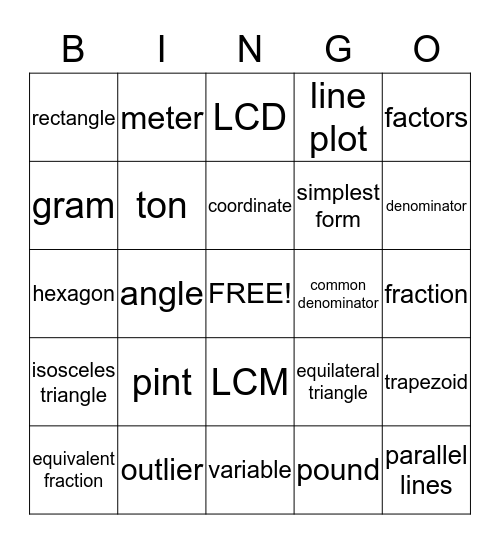# Math VocabularyThis bingo card has a free space and 56 words: area, equivalent fraction, volume, rectangle, variable, quotient, ton, obtuse angle, simplest form, decimal, line plot, cubed, prime number, hundredth, gallon, mixed number, factors, angle, order of operations, parallel lines, common denominator, perimeter, equilateral triangle, LCD, acute triangle, coordinate, cubic unit, cup, denominator, divisable, equation, equivalent decimal, exponent, fluid ounce, fraction, gram, hexagon, improper fraction, isosceles triangle, meter, LCM, liter, multiple, numerator, parallelogram, pint, outlier, line plot, obtuse triangle, polygon, pound, right angle, rhombus, scalene triangle, trapezoid and whole number.

⚠ This card has duplicate items: line plot (2)

## Play Online

Share this URL with your players:For more control of your online game, create a clone of this card first.

## Probabilities

With players vying for a you'll have to call about __ items before someone wins. There's a __% chance that a lucky player would win after calling __ items.

Tip: If you want your game to last longer (on average), add more unique words/images to it.